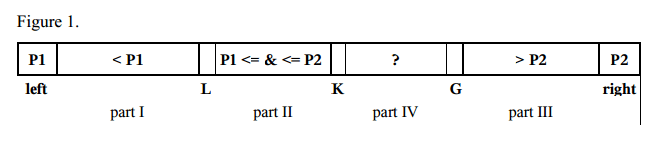## Dual-Pivot Quicksort

Notice:

• the Dual-Pivot Quicksort by Vladimir Yaroslavskiy, Jon Bentley, and Joshua Bloch is different als 3-Way-Quicksort.
• This Dual-Pivot Quicksort is build in Java API above V7 as default algorithm for sorting arrays of primitives.
• The Default algorithm for sorting arrays of objects in Java API above V7 is Timsort.

In the java doc.

The sorting algorithm is a Dual-Pivot Quicksort by Vladimir Yaroslavskiy, Jon Bentley, and Joshua Bloch. This algorithm offers O(n log(n)) performance on many data sets that cause other quicksorts to degrade to quadratic performance, and is typically faster than traditional (one-pivot) Quicksort implementations.

In the google search result, Theory of quick sort algorithm:

1. Pick an element, called a pivot, from the array.
2. Reorder the array so that all elements, which are less than the pivot, come before the pivot and all elements greater than the pivot come after it (equal values can go either way). After this partitioning, the pivot element is in its final position.
3. Recursively sort the sub-array of lesser elements and the sub-array of greater elements.

In comparison,dual-pivot quick sort:1. For small arrays (length < 17), use the Insertion sort algorithm.
2. Choose two pivot elements P1 and P2. We can get, for example, the first element a[left] as P1 and the last element a[right] as P2.
3. P1 must be less than P2, otherwise they are swapped. So, there are the following parts:
• part I with indices from left+1 to L–1 with elements, which are less than P1,
• part II with indices from L to K–1 with elements, which are greater or equal to P1 and less or equal to P2,
• part III with indices from G+1 to right–1 with elements greater than P2,
• part IV contains the rest of the elements to be examined with indices from K to G.
4. The next element a[K] from the part IV is compared with two pivots P1 and P2, and placed to the corresponding part I, II, or III.
5. The pointers L, K, and G are changed in the corresponding directions.
6. The steps 4 – 5 are repeated while K ≤ G.
7. The pivot element P1 is swapped with the last element from part I, the pivot element P2 is swapped with the first element from part III.
8. The steps 1 – 7 are repeated recursively for every part I, part II, and part III.

Note : the content of this post is reposted from the following mentioned repost source.

Reference: